# Case Based Questions ChemistryCase-Based Questions Chemistry

Solid State                                 Passage – 1

A lattice can be generated by repeating a small portion called the unit cell. A crystal structure is made of atoms. In other words, the structure is an ordered array of atoms, ions or molecules. The different type of unit cells are Primitive Cubic Unit Cell, Body-centered Cubic Unit Cell, Face centered cubic unit cell.
The primitive cubic unit cell has atoms only at its corner. Each atom at a corner is shared between eight adjacent unit cells four unit cells in the same layer and four-unit cells of the upper or lower layer. Therefore, only 1/8th of an atom actually belongs to a particular unit cell. A body-centered cubic unit cell has an atom at each of its corners and also one atom at its body centre. A face-centered cubic unit cell contains atoms at all the corners and at the centre of all the faces of the cube. The atom present at the face-center is shared between 2 adjacent unit cells and only 1/2 of each atom belongs to an individual cell.

(a). What are the characteristics of a Unit cell?
Ans (a). (i) The dimensions of the unit cell along three edges: a, b and c.
(ii) The angles between the edges: α (between b and c); β (between a and c) and γ (between a and b).

(b). Give the significance of a lattice point.
Ans (b). The lattice point denotes the position of a particular constituent in the crystal lattice. It may be an atom, ion, or molecule. The arrangement of the lattice points in space is responsible for the shape of a particular crystalline solid.

(c). What is the number of atoms in a unit cell of a face-centered cubic crystal?
Ans (c). 8 corners × 1/8 per corner atom = 8 × 1/8 = 1 atom
6 face-centred atoms × 1/2 atom per unit cell = 3 atoms
Therefore the total number of atoms in a unit cell = 4 atoms.

(d). A solid with cubic crystal is made of two elements P and Q. Atoms of Q are at the corners of the cube and P at the body-centre. What is the formula of the compound?
Ans (d).  As atoms of Q are present at the 8 centres of the cube, therefore, number of atoms of Q in the unit cell = 8 × 1/8 = 1 atom
The atom P is at the body centre Number of atoms = 1
The ratio of atoms P:Q = 1:1
Hence, the formula of the compound is PQ

Case Based Questions Chemistry

Passage – 2

Solids may be classified as amorphous and crystalline. Amorphous solids are isotropic whereas crystalline solids are anisotropic. Solids can also be classified on the basis of the type of forces of attraction as ionic, covalent, metallic, and molecular solids. Unit cells represent crystal lattice. Types of crystal lattice-bcc, fcc, simple cubic, end centered cubic are most common in seven crystal systems and 14 bravais lattices. The constituent particles arrange in square close packing, bcc, fcc, hcp. Packing efficiency of hcp and fcc is 74%, bcc 68%, and simple cubic packing (52%). The vacant sites in packing are called voids, tetrahedral, and octahedral being most common. The density of solids can be calculated by using.

There are defects in the crystal lattice, point defects like Frenkel and Schottky. Non-stoichiometric defects are metal excess type and metal deficient type and impurity defects. These defects affect the properties of solids. Solids may be paramagnetic, diamagnetic, ferromagnetic, ferrimagnetic depending upon the presence of unpaired electrons. Solids may be conductors, insulators, semiconductors (n-type and p-type) formed by doping (adding group 13 or 15 as an impurity, band theory can explain conductors, semiconductors, and insulators.

(a). Which type of defects are shown by ZnS?
Ans (a). Frenkel Defect

(b) A given metal has an fcc structure with an edge length of 361 pm. What is the radius of an atom?
Ans (b).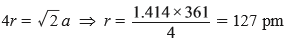(c) A compound is formed by cation ‘C’ which occupies 75% of octahedral voids and anion ‘A’ form hcp. What is the formula for the compound?
Ans (c). C3A4

(d) What is a forbidden zone?
Ans (d). The energy gap between the conduction band and valence band is large in insulators called the forbidden zone.

(e) Give one ferrimagnetic mixed oxide.
Ans (e).  Fe3O4 is ferromagnetic.

(f) What type of solid is silicon carbide (Carborundum)?
Ans (f). Covalent Solid.

Case Based Questions Chemistry

Solutions:                                           Passage – 1

The solutions which obey Raoult’s law over the entire range of concentration are known as the ideal solution. However, there are many solutions that do not obey Raoult’s law, such solutions are called non-ideal solutions. They show deviation from Raoult’s law i.e. ideal behavior which may be positive or negative. The solution of ethanol and acetone, chloroform and acetone, etc. falls into this category.

(a) State Raoult’s law.
Ans (a). Raoult’s Law states that the vapour pressure of an ideal solution is proportional to the mole fraction of the solvent.

(b) What type of deviation from Raoult’s law is shown by a mixture of ethanol and acetone and why?
Ans (b). mixture of ethanol and acetone shows a positive deviation from Raoult’s Law. Pure ethanol possesses hydrogen bonding. Introduction of acetone between the molecules of ethanol results in the breaking of some of these hydrogen bonds. Due to the weakening of interactions, the solution shows a positive deviation from Raoult’s law.

(c) What type of deviation from Raoult’s law is shown by a solution of chloroform and acetone and why?
Ans (c). Chloroform and acetone shownegative deviation from Raoult’s law. This is because there is an attractive interaction between these two that results in the formation of hydrogen bonding.

(d) Give two examples of solutions that are nearly ideal in behavior.
Ans (d). (i) Benzene and Toluene
(ii) n-Heptane and n-Hexane

Case Based Questions Chemistry

Passage – 2

Solutions are a homogeneous mixture of two or more substances. The ideal solution follows Raoult’s law. The vapour pressure of each component is directly proportional to their mole fraction if both solute and solvent are volatile. The relative lowering of vapour pressure is equal to the mole fraction of solute if the only solvent is volatile. Non-ideal solution form azeotropes which cannot be separated by fractional distillation. Henry’s law is a special case of Raoult’s law applicable to gases dissolved in liquids.
Colligative properties depend upon a number of particles of solute. Relative lowering of vapour pressure, elevation in boiling point, depression in freezing point, and osmotic pressure are colligative properties that depend upon mole fraction of solute, morality, and molarity of solutions. When solute undergoes either association or dissociation, molecular mass determined by a colligative property will be abnormal. van’t Hoff factor is used in such cases which is the ratio of normal molecular mass over observed molar mass.

(a) 50 ml of an aqueous solution of glucose (Molar mass 180 g/mol) contains 6.02 × 1022 molecules. What is molarity?
Ans (a).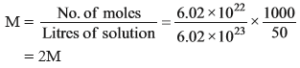(b) Identify which liquid has a lower vapour pressure at 90°C if the boiling points of liquid ‘A’ and ‘B’ are 140°C and 1800C respectively.
Ans (b). ‘B’ will have lower vapour pressure because its boiling point is higher.

(c) What type of azeotropes are formed by non-ideal solution showing negative deviation from Raoult’s law?
Ans (c). Maximum boiling azeotropes.

(d) For a 5% solution of area (molar mass 60 g mol–1), calculate the osmotic pressure at 300 K (R = 0.0821 L atm k–1).
Ans (d).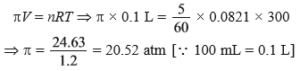(e) Predict the van’t Hoff factor (i) CH3COOH dissolved in water, (ii) dissolved in benzene.
Ans (e) (i) i > 1, because dissociation takes place.
(ii) i < 1, because association takes place.

(f) Why meat is preserved for a longer time by salting?
Ans (f). Salt inhibits the growth of microorganisms by drawing out water from microbial cells through osmosis 20% salt is needed to kill most species of unwanted bacteria.

(g) Why 0.1 M KCl has a higher boiling point than 0.1 M glucose solution?
Ans (g). It is because KCl dissociates into ions, it has double particles as compared to glucose. Therefore, elevation in boiling point is double.

Case Based Questions Chemistry

Passage – 3

Solution plays a very important role in our daily life. Alloys, a homogeneous mixture of metal are solutions of solid in solid. 1 ppm (parts per million) of fluoride ions prevent tooth decay. All intravenous injections must be isotonic with our body fluids, i.e. should have the same concentration as blood plasma. Diabetic patients are more likely to have a heart attack and high blood pressure due to the higher glucose levels in the blood. Common salt increases blood pressure because Na+ mixes up with blood. Aquatic species are more comfortable in cold water than warm water.

(a) 0.1 M glucose is not isotonic with 0.1 M KCl solutions. Why?
Ans (a). This is because they do not have the same osmotic pressure as the number of particles is different.

(b) A solution contains 5.85 g of NaCl (molar mass 58.5 g mol–1) per litre of the solution, has osmotic pressure 4.75 atm of 27°C. Calculate the degree of dissociation of NaCl in this solution.  [R = 0.0821 LatmK–1mol–1].
Ans (b).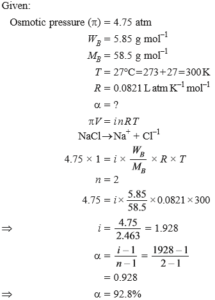(c) What will happen if blood cells are placed in saline water (hypertonic solution)?
Ans (c). These will shrink.

(d) Calculate the molarity of ethanol solution in which mole fraction of water is 0.88.
Ans (d).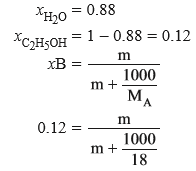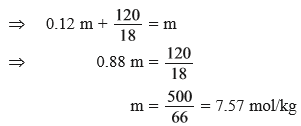(e) What will happen if the pressure applied on the solution side is more than the osmotic pressure when solvent and solution are separated by a semipermeable membrane?
Ans (e). The process of reverse osmosis will take place. It is used for the desalination of water.

Case Based Questions Chemistry

Case Based Questions Chemistry